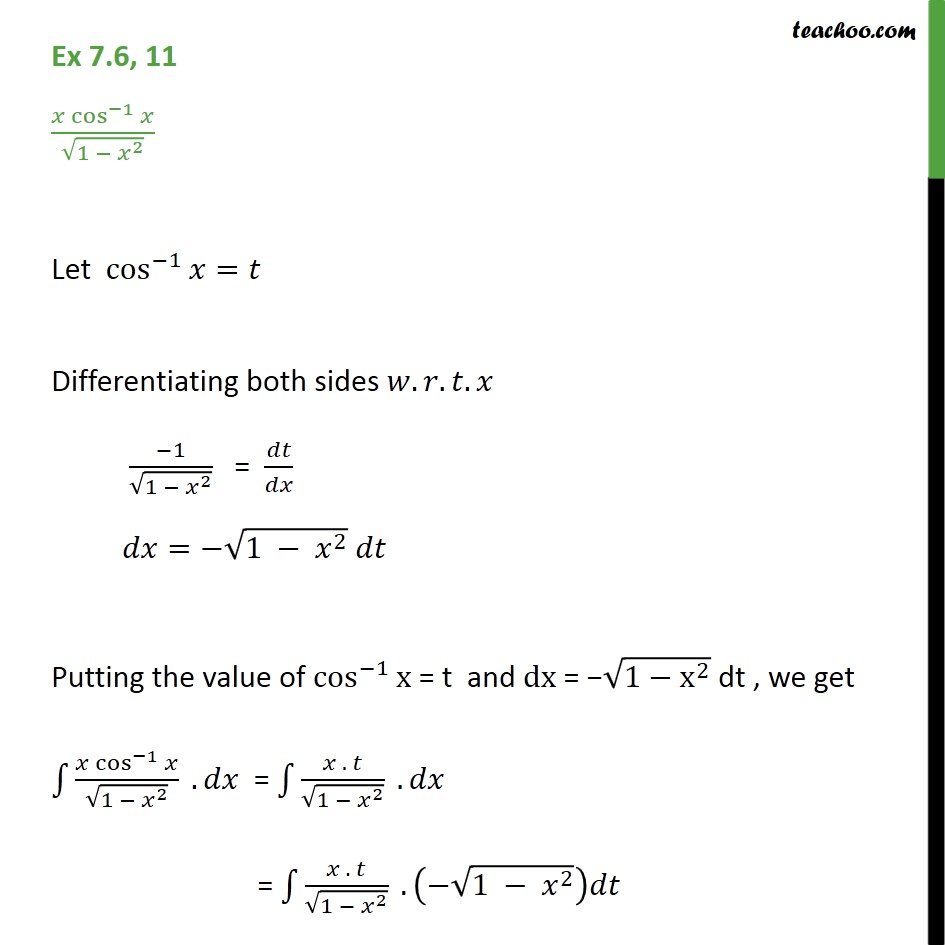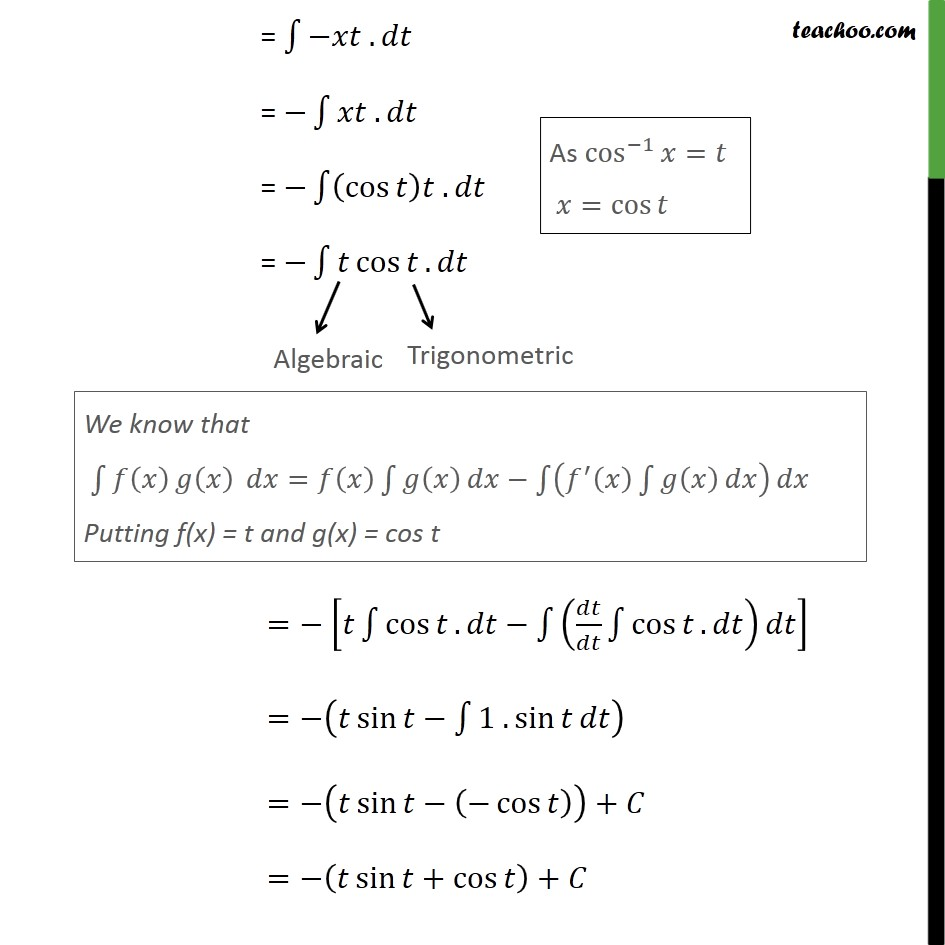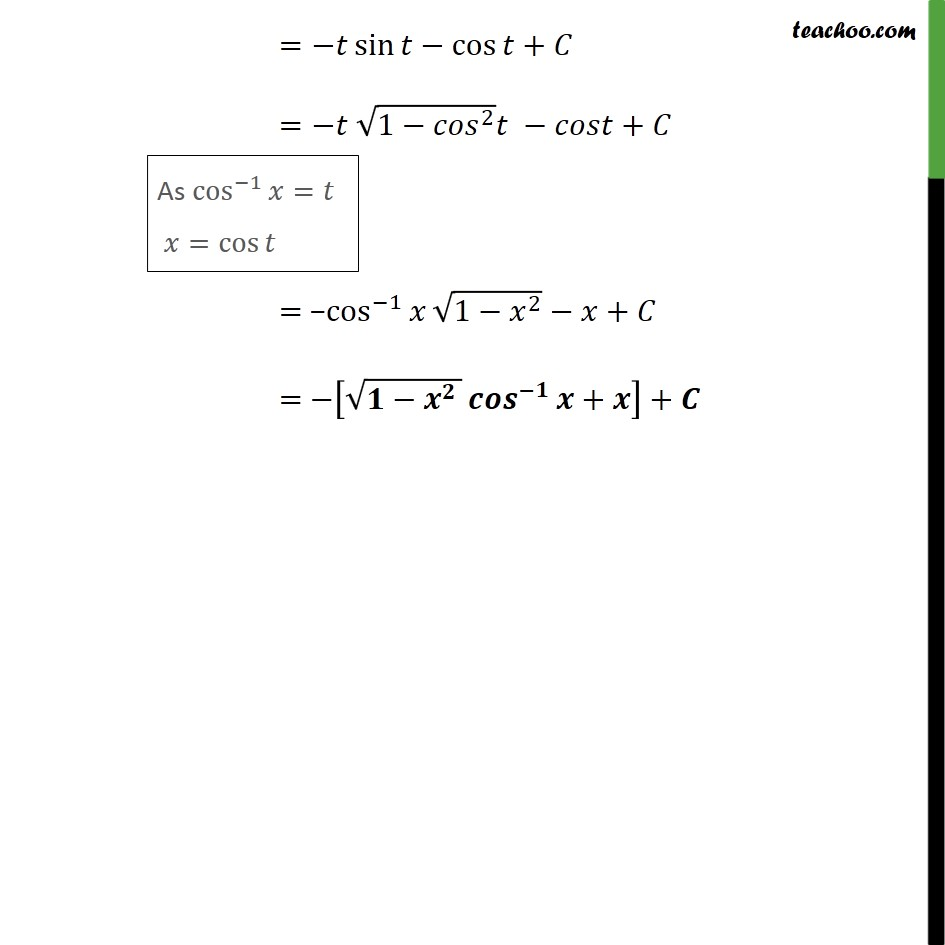Integration by parts

Chapter 7 Class 12 Integrals
Concept wiseIntroducing your new favourite teacher - Teachoo Black, at only ₹83 per month

### Transcript

Ex 7.6, 11 𝑥 cos﷮−1﷯﷮𝑥﷯﷮ ﷮1 − 𝑥﷮2﷯﷯﷯ Let cos﷮−1﷯﷮𝑥﷯=𝑡 Differentiating both sides 𝑤.𝑟.𝑡.𝑥 −1﷮ ﷮1 − 𝑥﷮2﷯﷯﷯ = 𝑑𝑡﷮𝑑𝑥﷯ 𝑑𝑥=− ﷮1 − 𝑥﷮2﷯﷯ 𝑑𝑡 Putting the value of cos﷮−1﷯﷮x﷯ = t and dx = − ﷮1− x﷮2﷯﷯ dt , we get ﷮﷮ 𝑥 cos﷮−1﷯﷮𝑥﷯﷮ ﷮1 − 𝑥﷮2﷯﷯﷯﷯ .𝑑𝑥 = ﷮﷮ 𝑥 . 𝑡﷮ ﷮1 − 𝑥﷮2﷯﷯﷯﷯ .𝑑𝑥 = ﷮﷮ 𝑥 . 𝑡﷮ ﷮1 − 𝑥﷮2﷯﷯﷯﷯ . − ﷮1 − 𝑥﷮2﷯﷯﷯𝑑𝑡 = ﷮﷮−𝑥𝑡 . 𝑑𝑡﷯ = − ﷮﷮𝑥𝑡 . 𝑑𝑡﷯ = − ﷮﷮ cos﷮𝑡﷯﷯𝑡 . 𝑑𝑡﷯ = − ﷮﷮𝑡 cos﷮𝑡﷯. 𝑑𝑡﷯ =− 𝑡 ﷮﷮ cos﷮𝑡﷯. 𝑑𝑡﷯− ﷮﷮ 𝑑𝑡﷮𝑑𝑡﷯ ﷮﷮ cos﷮𝑡﷯. 𝑑𝑡﷯﷯𝑑𝑡﷯﷯ =− 𝑡 sin﷮𝑡﷯− ﷮﷮1 . sin﷮𝑡﷯𝑑𝑡﷯﷯ =− 𝑡 sin﷮𝑡﷯− − cos﷮𝑡﷯﷯﷯+𝐶 =− 𝑡 sin﷮𝑡﷯+ cos﷮𝑡﷯﷯+𝐶 =−𝑡 sin﷮𝑡﷯− cos﷮𝑡﷯+𝐶 =−𝑡 ﷮1− 𝑐𝑜𝑠﷮2﷯﷯𝑡 −𝑐𝑜𝑠𝑡+𝐶 = − cos﷮−1﷯﷮𝑥﷯ ﷮1− 𝑥﷮2﷯﷯−𝑥+𝐶 = − ﷮1− 𝑥﷮2 ﷯﷯ cos﷮−1﷯﷮𝑥﷯+𝑥﷯+𝐶